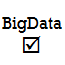# Converting Z Score to SQL/HQLThe Z Score A.K.A Standard Score is a statistical measurement of a score’s relationship to the baseline of a group. In this post I convert it to SQL, specifically Hive’s HQL.

I was calculating the Z Score for product trend analysis and thought I’d share my work at converting this statistical measurement to SQL. The example that I provide is for an old version of Hive Query Language/HQL, so there are more optimal ways to write it if your SQL has a standard deviation function.$z=(x - \mu )/\sigma$

z = (observation – average) / stddev

Z score is good for machine learning scenarios because it supports a running total by storing total values, total values², and the number of observations.

You can see in the SQL here that I manually calculate the standard deviation because of the lack of a stddev() function in the version of Hive that I’m using.

(a.total – (a.total + COALESCE(b.total,0) / 2)) / sqrt(COALESCE(b.sqtotal, 0) + POW(a.total, 2) / DATEDIFF(‘$today’, ‘2015-10-30’) – POW((a.total + COALESCE(b.total,0) / DATEDIFF(‘$today’, ‘2015-10-30’)), 2))

If you want to get meaningful results when analyzing trending product sales you will probably want to weight the Z score with any reduction in price of the product as well as the rank of the product by units sold versus the actual units sold.

I hope you find this article on calculating z-score using SQL useful.